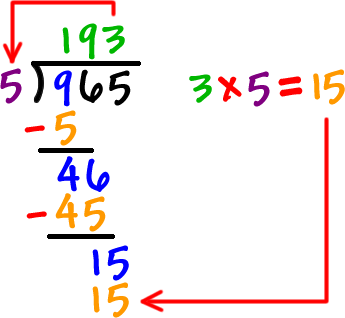Long Division
3 years ago
tejkej26
Save
Edit
Host a game
Live GameLive
Homework
Solo Practice
Practice10 QuestionsShow answers
• Question 1
60 secondsQ. 369 / 3 = x
123
129
133
111
• Question 2
60 seconds
Q. 46÷5=
7 r6
8 r4
8 r6
9 r4
• Question 3
60 seconds
Q. 46÷5=
7 r6
8 r4
8 r6
9 r4
• Question 4
60 seconds
Q. 68÷3=
21 r3
22 r2
22
21 r5
• Question 5
60 seconds
Q. 82÷6=
13 r4
12 r4
13
11 r8
• Question 6
60 seconds
Q. 39÷4=
10 r2
10 r3
9
9 r3
• Question 7
60 seconds
Q. 51÷5=
10 r1
10 r2
9 r3
9 r1
• Question 8
60 seconds
Q. 24÷2
5 r1
5
12
13
• Question 9
60 seconds
Q. 91÷7=
13 r3
13 r1
12
13
• Question 10
60 seconds
Q. 59÷5=